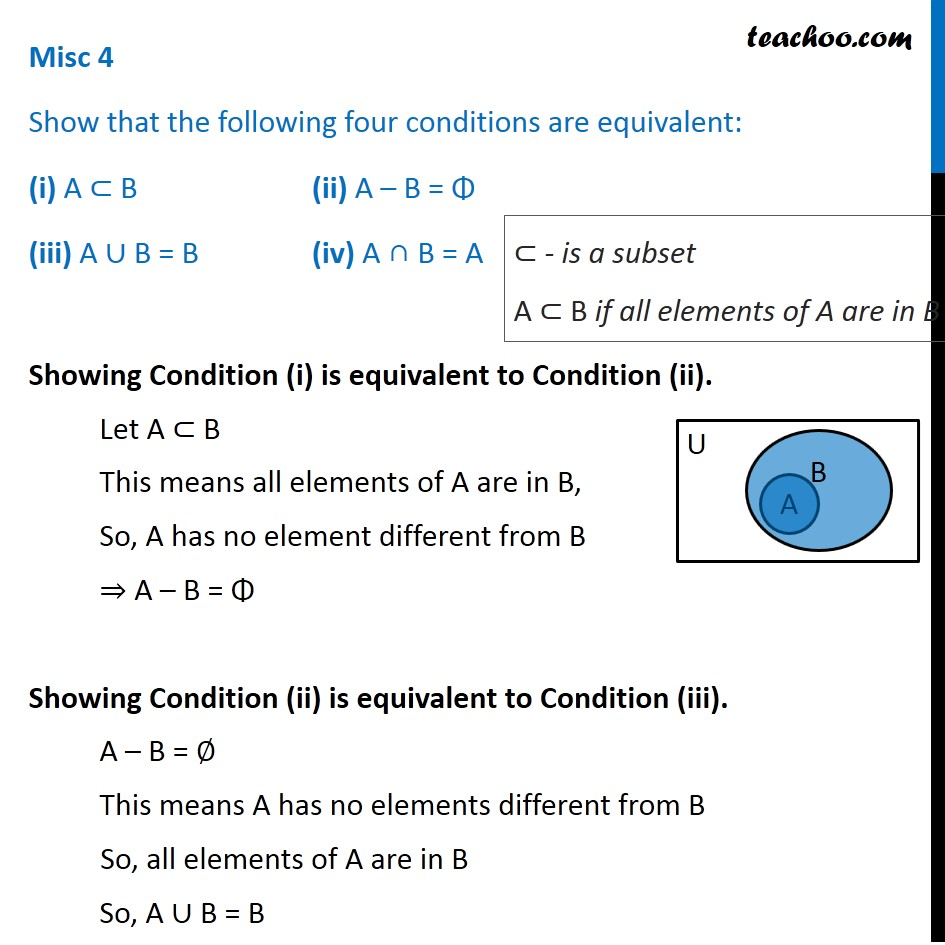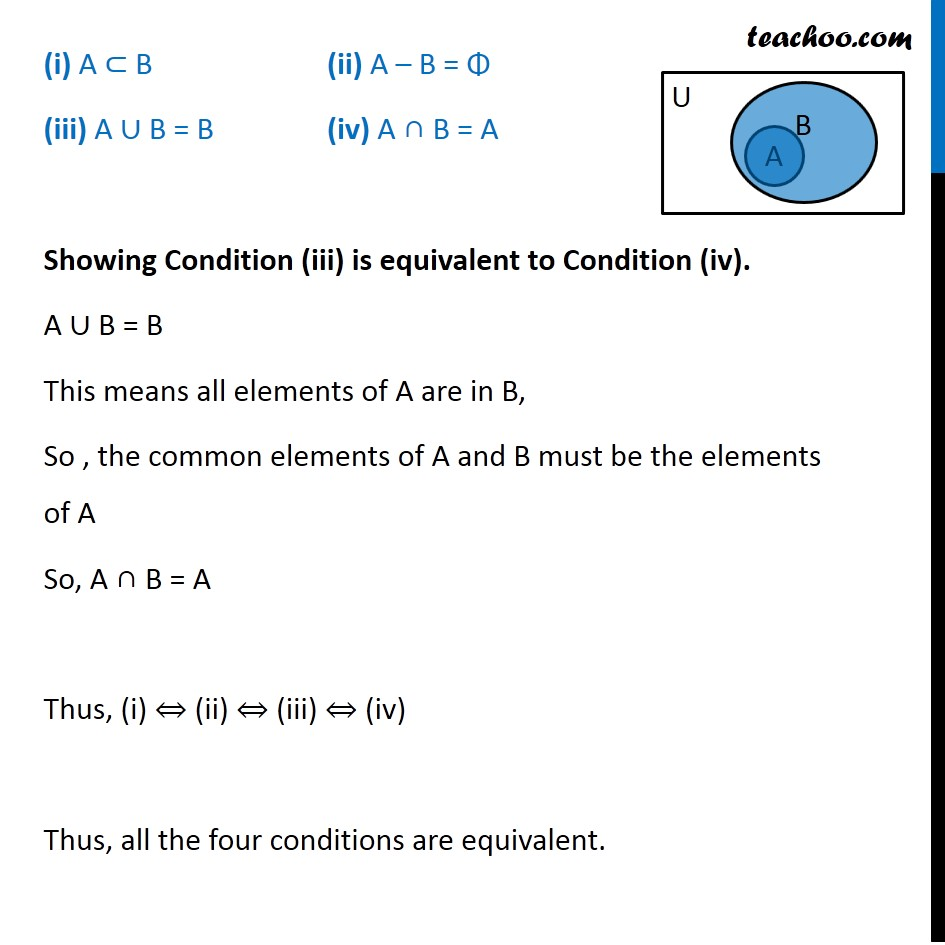Miscellaneous

Chapter 1 Class 11 Sets
Serial order wiseLearn in your speed, with individual attention - Teachoo Maths 1-on-1 Class

### Transcript

Misc 4 Show that the following four conditions are equivalent: (i) A ⊂ B (ii) A – B = Φ (iii) A ∪ B = B (iv) A ∩ B = A Showing Condition (i) is equivalent to Condition (ii). Let A ⊂ B This means all elements of A are in B, So, A has no element different from B ⇒ A – B = Φ Showing Condition (ii) is equivalent to Condition (iii). A – B = ∅ This means A has no elements different from B So, all elements of A are in B So, A ∪ B = B ⊂ - is a subset A ⊂ B if all elements of A are in B (i) A ⊂ B (ii) A – B = Φ (iii) A ∪ B = B (iv) A ∩ B = A Showing Condition (iii) is equivalent to Condition (iv). A ∪ B = B This means all elements of A are in B, So , the common elements of A and B must be the elements of A So, A ∩ B = A Thus, (i) ⇔ (ii) ⇔ (iii) ⇔ (iv) Thus, all the four conditions are equivalent.